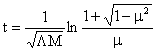(3)       The age of the universe

The outmost layer was expanded from   a0  to  a  in time  te ,  its equation is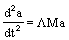or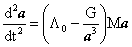(10)

Now put   L=constant,   upon solving this differential equation we obtain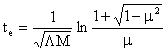The  te  is the minimum of expansion age from  Z=0   to  Z=2.3   because
L=
constant.   Substituting the value of cosmic parameter (Table 1), we get
te>18.6×10 years

Upon solving equation (10) or formula (14) can be written as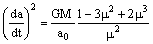using   a0=ma  to find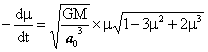thus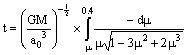Assuming   m=0.4   to be starting point of time, i.e.  t=0, we obtain
age curve in Fig.3.

Initial velocity of outmost layer equals zero to be impossible. If there
was Initial velocity about thousands of kilometer, this corresponded to the
beginning of repulsion expansion from  m=0.98.

Fig.5 Age curve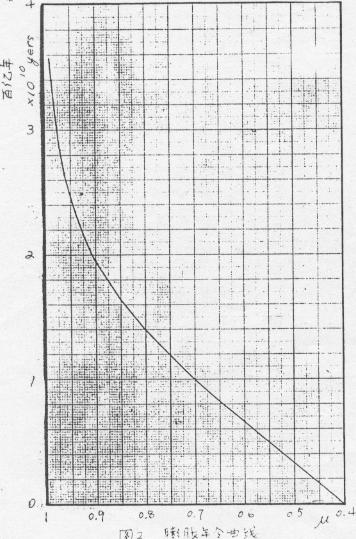_________________________________________________________

[note]

put  L=constant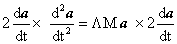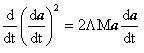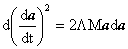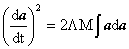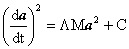When a=a0,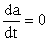, thus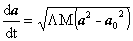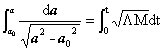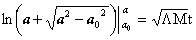Substituting  a0=μa ,  we  get